Letters for Digits - Multiplication Questions

Chapter 16 Class 8 Playing with Numbers
Concept wise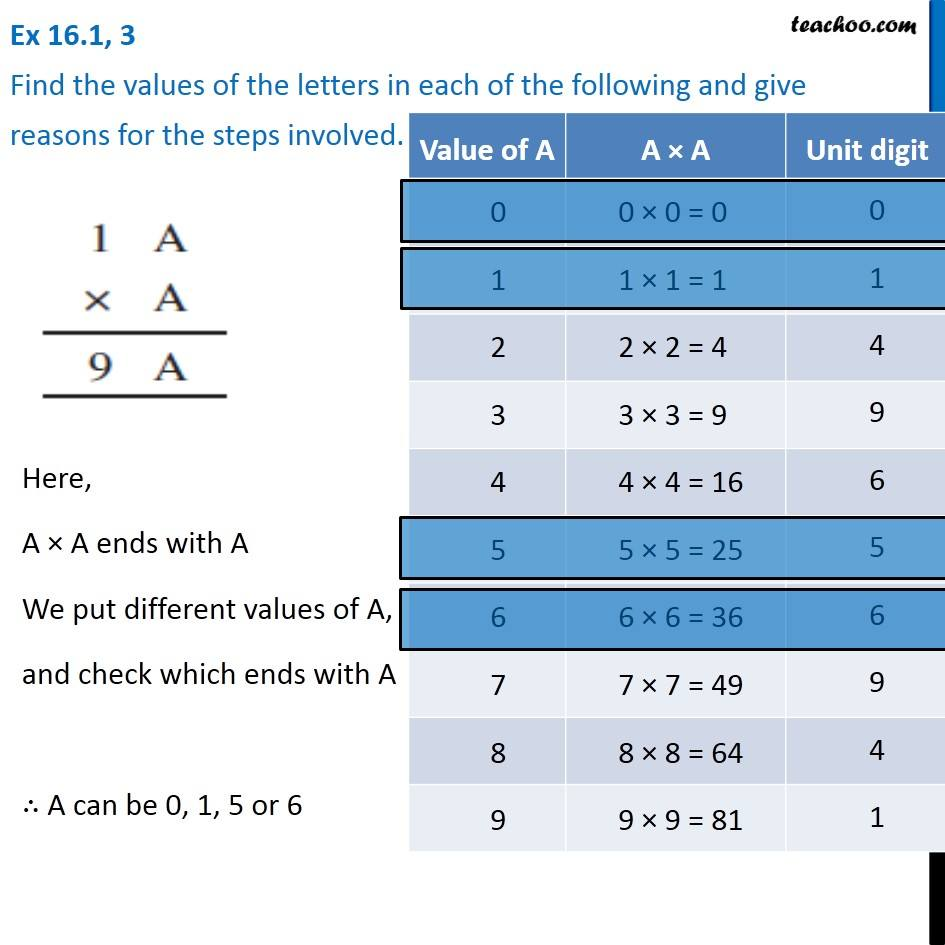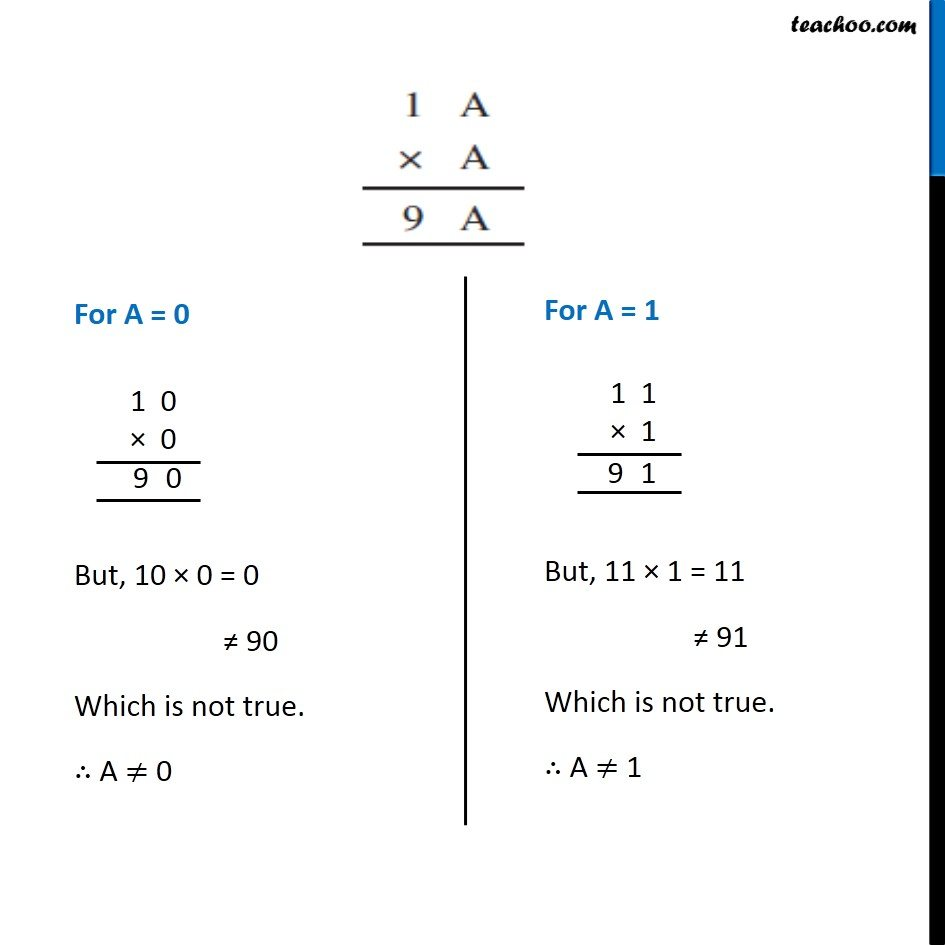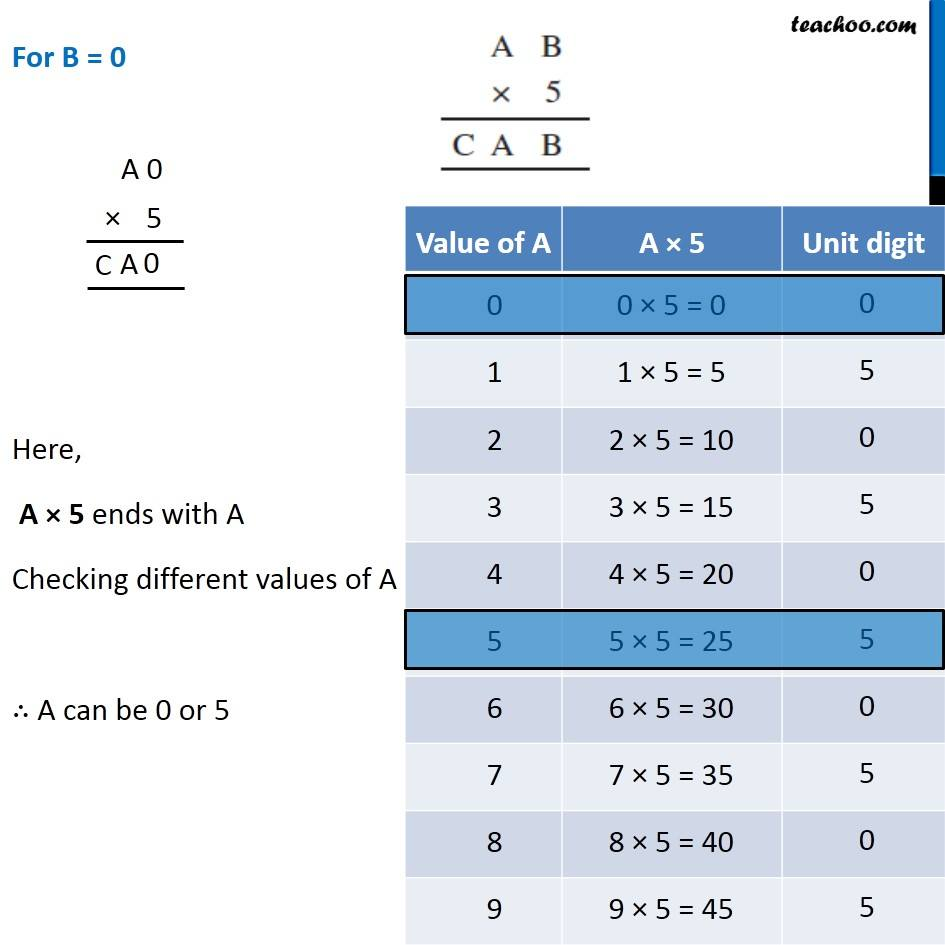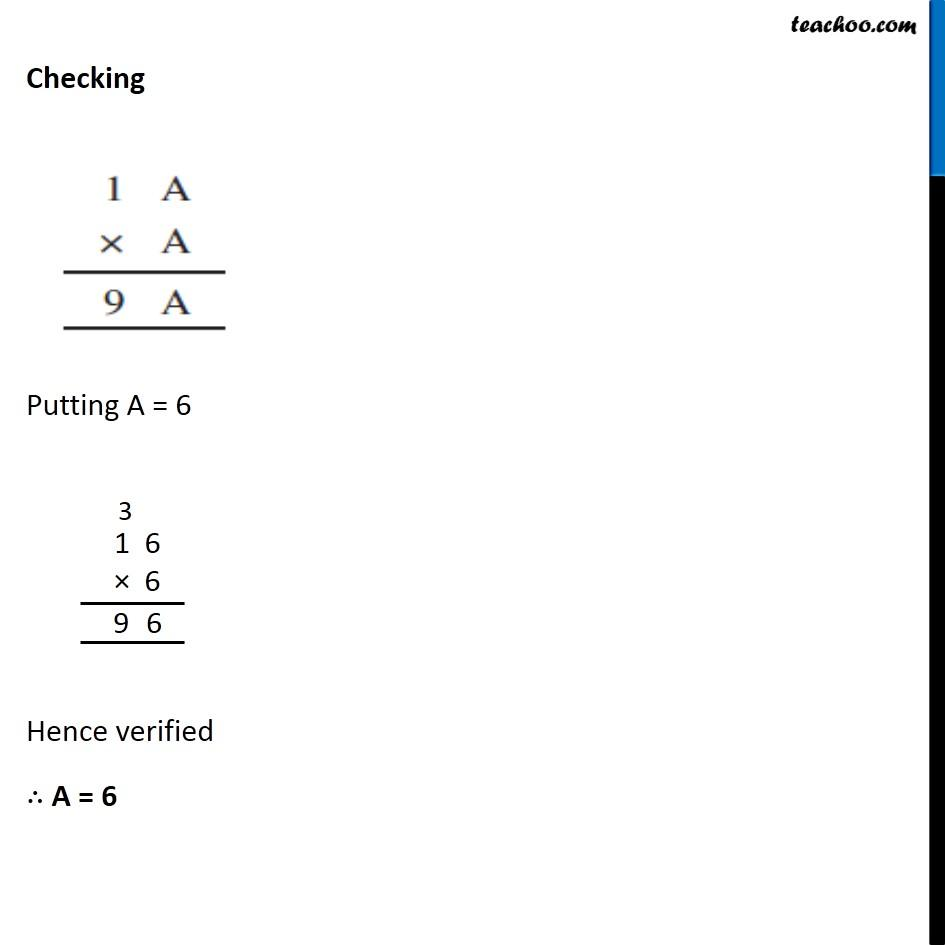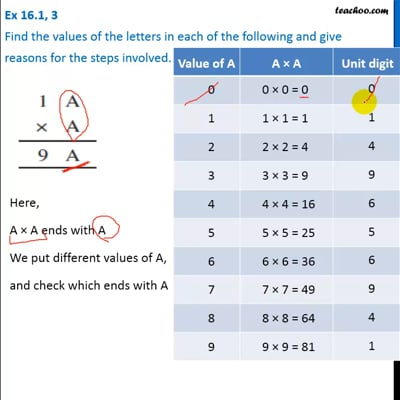This video is only available for Teachoo black users

Introducing your new favourite teacher - Teachoo Black, at only ₹83 per month

### Transcript

Ex 16.1, 3 Find the values of the letters in each of the following and give reasons for the steps involved. Here, A × A ends with A We put different values of A, and check which ends with A ∴ A can be 0, 1, 5 or 6 For A = 0 But, 10 × 0 = 0 ≠ 90 Which is not true. ∴ A ≠ 0 For A = 1 But, 11 × 1 = 11 ≠ 91 Which is not true. ∴ A ≠ 1 For A = 1 But, 11 × 1 = 11 ≠ 91 Which is not true. ∴ A ≠ 1 For A = 5 But, 15 × 5 = 75 ≠ 95 Which is not true. ∴ A ≠ 5 For A = 6 Here, 16 × 6 = 96 Which is true. ∴ A = 6 Checking Putting A = 6 Hence verified ∴ A = 6